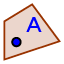# Distance Formula Practice

Interactive practice using distance formula.

## Write the distance formula down

Suppose you three friends who live at location C, D and E. You want know how many miles you will travel to visit from your house at location A. a). Move point B to C. What is the distance? b). Move point B to D. What is the distance? C). Move point B to E. What is the distance?

Check all that apply

Name the imageName the imageName the image